Scheduling problems – problems that seek to order a sequence of tasks while preserving an order of precedence – can be solved by performing a topological sort on a directed acyclic graph (DAG). Certainly, we can review the DAG and the topological sorting algorithms. But that does not go deep enough and it does not fully explore the mechanisms behind why a DAG paired with a topological sort is an effective solution. To more thoroughly understand the problem and the solution, a deeper dive has to be taken—and that means understanding the mathematics behind it.

Order theory is the branch of mathematics that we will explore as we probe partial ordering, total ordering, and what it means to the directed acyclic graph and topological sort.

We will begin with a partial order and a partially ordered set and examine how these concepts are related to a directed acyclic graph. This will be followed by an examination of a total order and a totally ordered set. With this foundation, we will be able to tackle the topological sort and its usefulness in scheduling problems.

## Partial Order

A partial order, or partial ordering, is a relation between pairs of elements in a set. More specifically, it is a relation that is reflexive, transitive, and antisymmetric.

Given the set S {1, 2, 3}, the properties of reflexivity, transitivity, and antisymmetry can be explained using the ≤ relation as follows.

The reflexive property states that an element is equal, or comparable, to itself:

Reflexive Property
1 ≤ 1

The transitive property states that there is an order of precedence in a chain of elements:

Transitive Property
If 1 ≤ 2
And 2 ≤ 3
Then 1 ≤ 3

The antisymmetric property states that given a relation R, two elements cannot be related to each other if they are distinct elements. More formally, if aRb and a ≠ b, then bRa. But if aRb and a = b, then bRa.

Antisymmetric Property
If 1 ≤ 2
Then 2 ≰ 1

Because the ≤ relation satisfies the reflexive, transitive, and antisymmetric properties, it is a partial order on the set {1, 2, 3}.

Another common example of a partial order is alphabetical order. Replace the set above with the set {a, b, c} and you’ll find that an alphabetical order satisfies the properties of reflexivity, transitivity, and antisymmetry.

### The Poset

A partially ordered set, or poset, denoted as (S, R), is a set S with a partial order R. Let’s take our aforementioned set S and partial order R and denote it as the partially ordered set ({1, 2, 3}, ≤).

The items in this set are ordered under the relation aRb where a ≤ b. An example of this set contains the following items:

{(1, 1), (1, 2), (1, 3), (2, 2) (2, 3), (3, 3)}

Each ordered pair above represents one of the elements in the poset ({1, 2, 3}, ≤).

For example, the ordered pair (1, 1) illustrates the reflexive property of a partial order because 1 ≤ 1.

The ordered pair (1, 3) represents the transitive property of a partial order because 1 ≤ 2 and 2 ≤ 3, so 1 ≤ 3.

And lastly, because (2, 1), (3, 1), and (3, 2) are not elements of the set, the antisymmetric property is satisfied.

### The Partial in Partial Order

It is important to note that two elements a and b of a poset (S, ≼) are comparable if it is the case that a ≼ b or b ≼ a; otherwise, if neither is the case, a and b are incomparable. Here, the ≼ sign denotes a partial order.

In the poset ({1, 2, 3}, ≤), the partial order is the ≤ relation. Because for whatever a and b we choose from the elements {1, 2, 3}, it will be true that either a ≤ b or b ≤ a. For example, if a = 1 and b = 2, it is true that a ≤ b. So, it can be said that these elements, 1 and 2, from the set are comparable.

But if the poset contained a random letter as well, such as in the poset ({1, 2, 3, z}, ≤), it would be the case that the element z is incomparable with the other elements. Elements 1, 2, 3 are comparable with each other, but when a = z or b = z, it is not true that a <= b or b <= a; therefore, z is an incomparable element in the poset because you cannot relate a letter to a number using the ≤ relation.

This is important to note because a partially ordered set is considered partially ordered; that is, every element within the set does not have to be comparable and there can exists incomparable elements within it.

When it is the case that every element is comparable, then the partially ordered set is considered a totally ordered set, and this will be discussed shortly.

## A DAG Is A Poset

Before continuing to discuss total orderings, I would like to offer some relevance for why the partially ordered set is being discussed alongside directed acyclic graphs and topological sorting.

Straightforwardly, a directed acyclic graph is a partially ordered set, which I can illustrate through an example.

#### An Example Through Dinner

Let’s say that I have planned out a simple dinner with my girlfriend, and I have a number of tasks that I need to complete. But I realize that some tasks depend on other tasks, so I have to finish some tasks before others. This has the potential to be a bit overwhelming to plan, but we do have graph theory and order theory at hand to make it much more manageable.

I first decide to determine every task that I have to do and collect them into a set T {A, B, C, D, E, F, G, H}.

T = {A, B, C, D, E, F, G, H} where
A = Buy Ingredients at Market
B = Make Guacamole
C = Get Gas
D = Buy Beer at Liquor Store
E = Open First Beer (While I Cook)
F = Boil Potatoes
G = Make Taquitos de Papas y Queso
H = Enjoy Dinner with Girlfriend

Having established which tasks I need complete, I begin to determine an order of precedence because, as I recall, I cannot start task A or D until I complete task C, and I definitely cannot start E until I complete those. I come up with a partially ordered set ({A, B, C, D, E, F, G, H}, <) where < represents a partial order relation based on my assessment of precedence. The contents of this poset is the following:

{(A, E), (B, H), (C, A), (C, D), (D, E), (E, B), (E, F), (F, G), (G, H)}

Reading the contents of the poset in set-builder notation makes it a little difficult to read and understand the full order of precedence of the tasks I need to complete. So, I can make a graph of the tasks and create directed edges using the ordered pairs in the poset. This produces the following graph: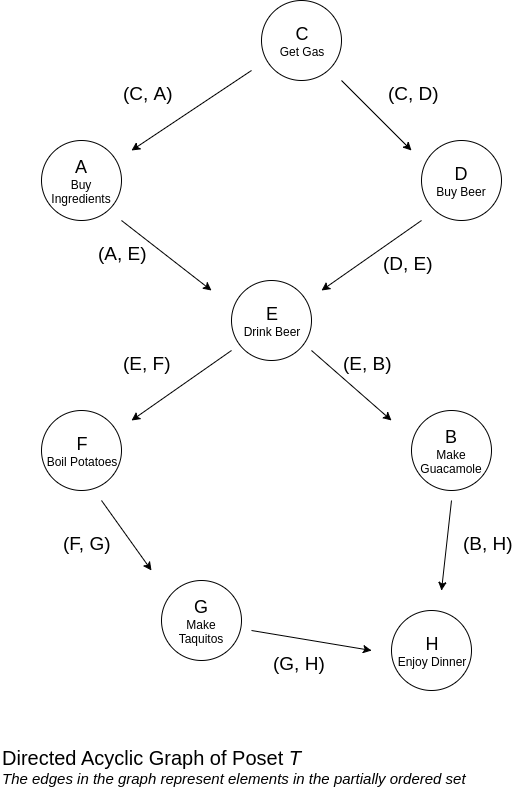This helps illustrate that a directed acyclic graph is a partially ordered set. The graph contains nodes for each distinct task, and the directed edges are created from the elements of the poset. Closer examination also shows that there are no cycles prsent.

While an illustration of the graph makes it clear that there is an order of precedence to the tasks, it still does not provide us with a schedule that preserves this precedence; that is, we do not quite know in which exact order we should complete the tasks. For that, we need to understand total ordering and the topological sort.

## Total Ordering

A total order, or linear order, is relation on a set similar to a partial order but with the added property of totality.

The property of totality states that every two elements from the poset (S, ≼) must be comparable. Remember that for two elements a and b to be comparable, it has to be the case that a ≼ b or b ≼ a.

Whereas a partial order may contain elements that are incomparable, the property of totality makes it so that every element within the set must be comparable to be considered as a totally ordered set. As a result, a totally ordered set is much more strict and produces a linear order compared to a partial order. This can be illustrated when you consider the poset (ℤ, <). This total order on the set of integers will produce a number line of the integers where it will be the case that a < b or b < a for any a, b Є ℤ.

We can illustrate this on a smaller, finite set, such as the totally ordered set ({-2, -1, 0, 1, 2}, <), which contains the following elments:

{(-2, -1), (-1, 0), (0, 1), (1, 2)}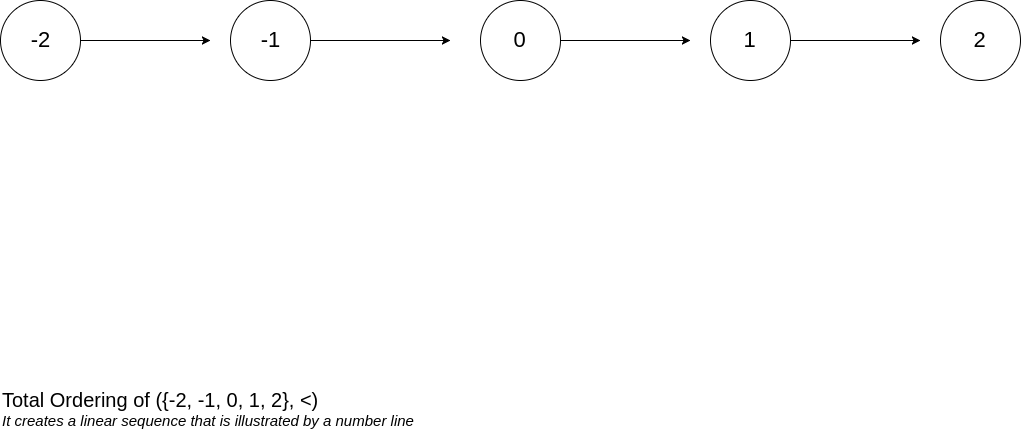## A Total Ordering of a Poset

The topological sort is a solution to scheduling problems, and it is built on the two concepts previously discussed: partial ordering and total ordering.

At its core, a topological sort, or a linear extension, is a total ordering of a partially ordered set.

We can arrive at this definition of a topological sort by first recalling what a directed acyclic graph is: it is a partial ordering of a set. In other words, a DAG is a partially ordered set, such as poset T ({A, B, C, D, E, F, G, H}, ≤) discussed earlier.

Again, recall the contents of this poset:

{(A, E), (B, H), (C, A), (C, D), (D, E), (E, B), (E, F), (F, G), (G, H)}

Performing a total ordering of the poset T, we can produce the following totally ordered set, which is one of many totally ordered sets:

{(C, A), (C, D), (A, E), (E, F), (F, G), (E, B), (B, H)}

The above totally ordered set can be distilled to clarify the following path through the graph:

C → A → D → E → F → G → B → H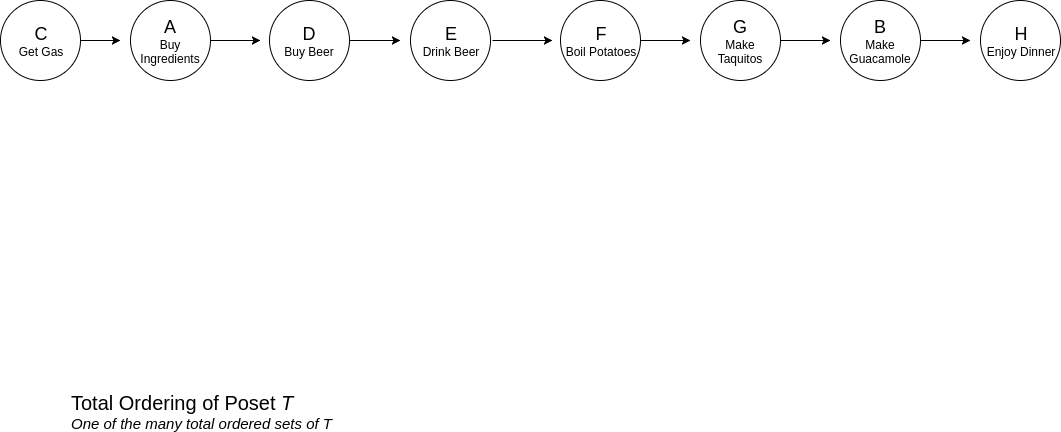And with this total ordering of the partially ordered set T, we have a valid schedule of tasks that preserve the order of precedence.

#### Multiple Valid Totally Ordered Sets

Note that there can be a number of different totally ordered sets. For example, another valid totally ordered set for poset T is the following:

{(C, D), (C, A), (D, E), (E, B), (E, F), (F, G), (G, H)}

Which produces the following schedule of tasks:

C → D → A → E → B → F → G → H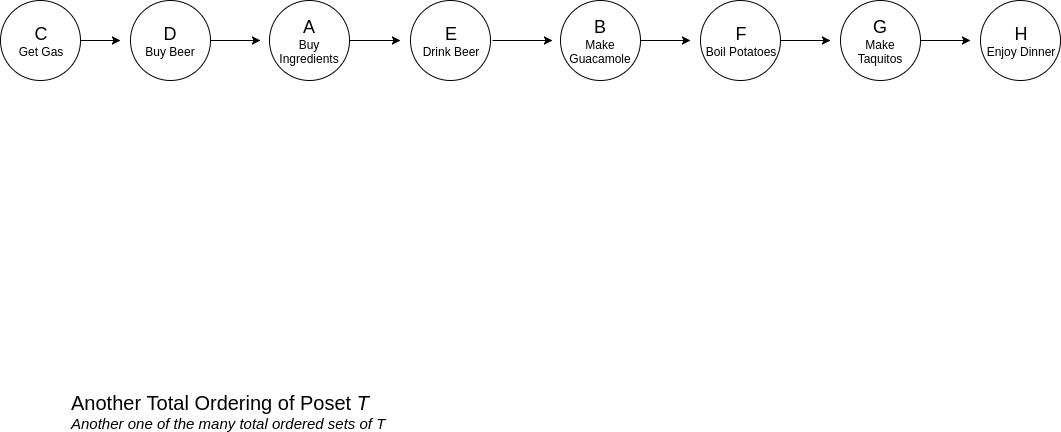There can be a number of different topological orderings of a directed acyclic graph, but identifying them all has the potential to be very inefficient, as identifying and counting them all can grow exponentially to the size of the graph.

## Topological Sorting Algorithms

The two common topological sorting algorithms are Kahn's algorithm and a modified depth-first search. Because I challened myself to be familiar with them, an overview of both of the algorithms will be discussed with code samples in three languages: Kahn's algorithm in Javascript and Java and the modified depth-first search in C#.

### Kahn's Algorithm

Kahn's algorithm can be reduced to 4 main task, detailed below:

1. Count and store the in-degrees of all nodes in a graph. The in-degree of a node A is the number of edges that have A as the target or destination.
2. Identify nodes with an in-degree of 0. Add each node to a set that will be iterated over and append each node to a list that will contain the sorted nodes.
3. While the set containing nodes with an in-degree of 0 is not empty, remove a node. For each node removed, iterate over its edges, decrementing the stored in-degree of each destination node in the edge. If a node now has an in-degree of 0, add it to the set and list per step 2.
4. Finally, return the list each node is appended to. This will contain a topological ordering of the nodes.

With minor adjustments to the input for my Javascript example, each code sample reads input from a file in the following format and with the following data, which reflects poset T in my example from earlier:

``````9
8
0 4
1 7
2 0
2 3
3 4
4 1
4 5
5 6
6 7
``````
1. The first line is the number E of edges in the graph.
2. The second line is the number N of nodes in the graph.
3. The last E lines are the edges, where the first number is the source node and the second number is the destination node.

### Javascript Example of Kahn's Algorithm

The Javascript example of Kahn's algorithm was constructed in three files for the sake of modularity.

First, I created a simple DirectedAcyclicGraph that just contains the bare-bones for what I needed:

``````"use strict";

module.exports = class DirectedAcyclicGraph {
constructor(size) {
}

setSize(size) {
this.Size = size;
}

}

}

}
``````

The next file contained Kahn's Algorithm which accepts as input an adjacency set of a graph and the size of a graph.

``````"use strict";

module.exports = function kahnsAlgorithm(adjacencySet, size) {

let noIncomingEdges = [];
let nodeInDegrees = {};
let pathString = "";

for (let n = 0; n < size; n++) {
nodeInDegrees[n] = 0;
}

for (let e = 0; e < adjacencySet.length; e++) {
for (let eI = 0; eI < edges.length; eI++) {
let destination = edges[eI];
nodeInDegrees[destination]++;
}
}

for (let c = 0; c < size; c++) {
if (!nodeInDegrees[c]) {
noIncomingEdges.push(c);
pathString += ` \${c} `;
}
}

while(noIncomingEdges.length) {
let currentNode = noIncomingEdges.shift();
if (!destinationEdges) { continue; }
for(let i = 0; i < destinationEdges.length; i++) {
let destination = destinationEdges[i];
nodeInDegrees[destination]--;
if (!nodeInDegrees[destination]) {
noIncomingEdges.push(destination);
pathString += ` \${destination} `;
}
}
}

return pathString;
}
``````

And lastly, the third file brings everything together. It directs Node to read from a file, construct the adjacency set, and call the function that performs Kahn's Algorithm:

``````"use strict";
const DirectedAcyclicGraph = require("./dag.js");
const kahnsAlgorithm = require("./kahnsAlgorithm.js");

const fs = require("fs");
const filePath = "../test.txt";

let numberOfNodes, numberOfEdges;
let testDag = new DirectedAcyclicGraph();

});

rl.on("line", (line) => {
if (line == "E") { numberOfEdges = line; }
else if(line == "N") {
numberOfNodes = line;
testDag.setSize(numberOfNodes);
}
else {
let source = line;
let destination = line;

}
});

rl.on("close", () => {
console.log("Path: ", path);
});
``````

Here is the screenshot of the console output: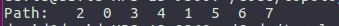This path, when translated to correspond with the nodes in poset T for the tasks in my dinner example, identifies the following valid topological path:

C → A → D → E → B → F → G → H

### Java Example of Kahn's Algorithm

The Java example is as follows:

``````public class Solution {
public static void main(String[] args) {
Scanner in;

try {
in = new Scanner(new File("blogTest.txt"));

int edges = in.nextInt();
int numOfNodes = in.nextInt();
HashMap<Integer, List<Integer>> adjacencySet = new HashMap<Integer, List<Integer>>();

for (int n = 0; n < numOfNodes; n++) {
}

in.nextLine();

for (int e = 0; e < edges; e++) {

Integer sourceNode = in.nextInt();
Integer destinationNode = in.nextInt();
}

System.out.println(path);

} catch(Exception e) {
System.out.println(e);
}
}

public static String KahnsAlgorithm(HashMap<Integer, List<Integer>> adjacencySet) {

HashMap<Integer, Integer> indegrees = new HashMap<Integer,Integer>();
String pathString = "";

indegrees.put(c, 0);
}

for (Integer c : e) {
Integer idegree = indegrees.get(c);
idegree++;
indegrees.replace(c,  idegree);
}

}

for(Entry<Integer, Integer> n : indegrees.entrySet()) {
if (n.getValue() == 0) {
pathString += n.getKey();
}
}

while(!noIncomingEdges.isEmpty()) {
Integer currentNode = noIncomingEdges.remove();
for (Integer destination : adjacencySet.get(currentNode)) {
Integer indegree = indegrees.get(destination);
indegree--;
indegrees.replace(destination, indegree);
if (indegree == 0) {
pathString += destination;
}
}
}

return pathString;
}
}

``````

Here is the screenshot of the output: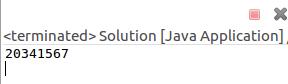The path, when translated, is the same as the one produced in the Javascript example:

C → A → D → E → B → F → G → H

### Modified Depth-First Search

A depth-first traversal of a graph begins at a node A and exhaustively explores each branch before exploring the next branch.

A depth-first search can be modified to produce a topological sorting of a directed acyclic graph by placing nodes on a stack after their children have been exhaustively explored.

Examine the diagram below which illustrates a topological sorting of Poset T using a depth-first search.

Green numbers indicate the position in which a node is visited.

Blue numbers indicate the position in which a node is placed on the stack.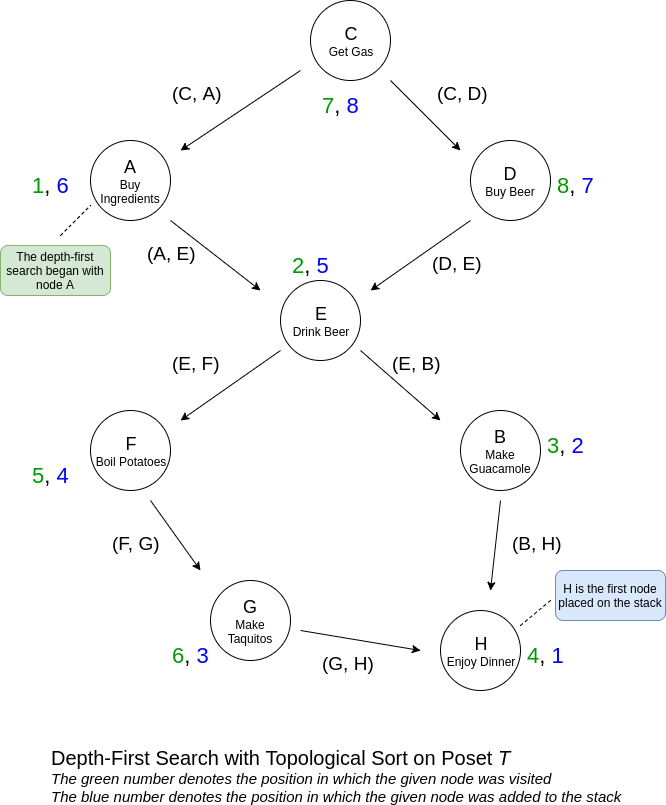The topological sorting algorithm begins on node A. A depth-first traversal on it moves onto E, since its the only child of A.

E has two children. At this point, the algorithm can move on to either child and still produce a valid topological sorting, as long as it exhaustively explores each child before moving on to the next.

In this instance, the algorithm goes through this first path before placing any nodes on the stack:

A → E → B → H

After reaching H, which has no children, the depth-first traversal goes back up, placing H and then B on the stack as the first two elements.

With one child fully explored, F, the other child of E is explored, which results in placing G and F on the stack.

Having explored all of the children of E, it is placed on the stack followed by A.

Lastly, C is explored, which leads to D, the only unexplored child of C. D and C are then placed on the stack.

The nodes in poset T are placed in this order on the stack:

H → B → G → F → E → A → D → C

When the nodes are popped off the stack, we get this valid topological sorting:

C → D → A → E → F → G → B → H

### C# Example of Depth-First Search Topological Sort

I created a DirectedAcyclicGraph class that contains the depth-first search topological sort algorithm:

`````` public class DirectedAcyclicGraph
{

public int Size = 0;

public DirectedAcyclicGraph(int size)
{
for (int i = 0; i < size; i++)
{
}

this.Size = size;
}

public void AddEdge(int source, int destination)
{
}

private void DFS(int node, int[] visitedNodes, Stack<int> stack)
{
visitedNodes[node] = 1;

for (int i = 0; i < destinationNodes.Length; i++)
{
int d = destinationNodes[i];
if (visitedNodes[d] == 0)
{
DFS(d, visitedNodes, stack);
}

}

stack.Push(node);
}

public string TopologicalSortDFS()
{
Stack<int> processedNodes = new Stack<int>();
char[] nodeLetters = new char[] { 'A', 'B', 'C', 'D', 'E', 'F', 'G', 'H' };

string path = "";

int[] visitedNodes = Enumerable.Repeat(0, this.Size).ToArray();

for (int i = 0; i < this.Size; i++)
{
if(visitedNodes[i] == 0)
{
DFS(i, visitedNodes, processedNodes);
}
}

while (processedNodes.Count > 0)
{
path += \$"{nodeLetters[processedNodes.Pop()]}  ";
}

return path;
}
}
``````

And here is the code that runs it:

``````public class Program
{
public static void Main(string[] args)
{
string path = "..\blogTest.txt";

try
{
if(File.Exists(path))
{
using (var fs = new FileStream(path, FileMode.Open, FileAccess.Read))
{
{

DirectedAcyclicGraph graph = new DirectedAcyclicGraph(numberOfNodes);

for (int i = 0; i < numberOfEdges; i++)
{
int source = int.Parse(line);
int destination = int.Parse(line);

}

string topologicalPath = graph.TopologicalSortDFS();

Console.WriteLine("Path through the Graph: " + topologicalPath);

}
}
}
}
catch (Exception e)
{
Console.WriteLine(e.Message);
}
}
}

``````

And here is a screenshot of the output: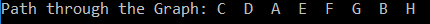## Conclusion

Scheduling problems are bound to be encountered because they are commonly found disguised in the problems and challenges we encounter in every day life. That is one of the reasons why I was immediately drawn to topological sorting.

Because every scheduling problem will vary, I will leave it up to the reader to decide on whether to use Kahn's algorithm or a modified depth-first search for their topological sorting needs. While my code examples are tailored to my specific example problem, implementations can nonetheless be derived from them to suit other scenarios.

### Afterthought

I learned a lot through self-study in this endeavor, so if any errors or inconsistencies are found, please feel free to point them out so that I can revise them. I would absolutely appreciate it :)